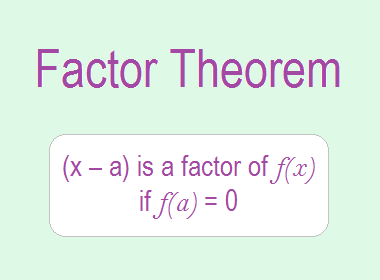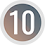# Factor Theorem## Factor Theorem

The factor theorem states that: “If f(x) is a polynomial and a is a real number, then (x – a) is a factor of f(x) if f(a) = 0.”

********************

10 Math Problems officially announces the release of Quick Math Solver, an Android App on the Google Play Store for students around the world.

********************

Proof:

If we divide f(x) by x – a, then we get Q(x) as quotient and R as remainder.

Then,

f(x) = (x – a).Q(x) + R ……..…. (i)

Putting x = a, then

f(a) = (a – a).Q(x) + R

or,     f(a) = R

When, f(a) = 0, then R = 0

Putting the value of R in (i) we get,

f(x) = (x – a).Q(x)

So, (x – a) is a factor of f(x).

Hence, (x – a) is a factor of f(x) if f(a) = 0.

Proved.

Corollary 1:

If f(x) is a polynomial and a is a real number, then (x + a) is a factor of f(x) if f(a) = 0.

Proof:

If we divide f(x) by x + a, then we get Q(x) as quotient and R as remainder.

Then,

f(x) = (x + a).Q(x) + R ……..…. (i)

Putting x = a, then

f(a) = (a + a).Q(x) + R

or,     f(a) = R

When, f(a) = 0, then R = 0

Putting the value of R in (i) we get,

f(x) = (x + a).Q(x)

So, (x + a) is a factor of f(x).

Hence, (x + a) is a factor of f(x) if f(a) = 0.

Proved.

Corollary 2:

If f(x) is a polynomial and a, b are real number, then (ax  b) is a factor of f(x) if f(b/a) = 0.

Proof:

If we divide f(x) by ax  b, then we get Q(x) as quotient and R as remainder.

Then,

f(x) = (ax  b).Q(x) + R ……..…. (i)

or,     f(x) = a(x – b/a).Q(x) + R

Putting x = b/a, then

f(b/a) = a(b/a – b/a).Q(x) + R

or,     f(b/a) = R

When, f(b/a) = 0, then R = 0

Putting the value of R in (i) we get,

f(x) = (ax  b).Q(x)

So, (ax  b) is a factor of f(x).

Hence, (ax  b) is a factor of f(x) if f(b/a) = 0.

Proved.

Corollary 3:

If f(x) is a polynomial and a, b are real number, then (ax + b) is a factor of f(x) if f(b/a) = 0.

Proof:

If we divide f(x) by ax + b, then we get Q(x) as quotient and R as remainder.

Then,

f(x) = (ax + b).Q(x) + R ……..…. (i)

or,     f(x) = a(x + b/a).Q(x) + R

Putting x = -b/a, then

f(b/a) = a(b/a + b/a).Q(x) + R

or,     f(b/a) = R

When, f(b/a) = 0, then R = 0

Putting the value of R in (i) we get,

f(x) = (ax + b).Q(x)

So, (ax + b) is a factor of f(x).

Hence, (ax + b) is a factor of f(x) if f(b/a) = 0.

Proved.

### Worked Out Examples

Example 1: Show that (x – 5) is a factor of 2x2 – 11x + 5.

Solution:

Here,

f(x) = 2x2 – 11x + 5

Comparing x – 5 with x – a, a = 5

Remainder R = f(5)

= 2×52 – 11×5 + 5

= 50 – 55 + 5

= 0

By factor theorem (x – 5) is a factor.

Example 2: Show that (x + 3) is a factor of 2x2 – x – 21.

Solution:

Here,

f(x) = 2x2 – x – 21

Comparing x + 3 with x – a, a = 3

Remainder R = f(–3)

= 2×(–3)2 – (–3) – 21

= 18 + 3 – 21

= 0

By factor theorem (x + 3) is a factor.

Example 3: Show that (2x – 1) is a factor of 2x2 – 11x + 5.

Solution:

Here,

f(x) = 2x2 – 11x + 5

Divisor 2x  1 can be written as 2(x  1/2) and comparing with x – a, a = 1/2

Remainder R = f(5)

= 2×(1/2)2 – 11×1/2 + 5

= 1/2 – 11/2 + 5

= 0

By factor theorem (2x – 1) is a factor.

Example 4: Show that (2x + 7) is a factor of 2x3 + 7x2 – 4x – 14.

Solution:

Here,

f(x) = 2x3 + 7x2 – 4x – 14

Divisor 2x + 7 can be written as 2(x + 7/2) and comparing with x – a, a = 7/2

Remainder R = f(–7/2)

= 2×(–7/2)3 + 7×(–7/2)2 – 4×(–7/2) – 14

= –343/4 + 343/4 + 14 – 14

= 0

By factor theorem (2x + 7) is a factor.

Example 5: If (x – 2) is a factor of the polynomial x2 – 3x + 5k, find the value of k.

Solution:

Here,

f(x) = x2 – 3x + 5k

Since (x – 2) is a factor of f(x) = x2 – 3x + 5k,

f(2) = 0

i.e.    22 – 3×2 + 5k = 0

or,     4 – 6 + 5k = 0

or,     –2 + 5k = 0

or,     5k = 2

or,     k = 2/5

Example 6: If (x + 1) and (x – 2) are factors of x3 + ax2 – bx – 6, find the values of a and b.

Solution:

Here,

f(x) = x3 + ax2 – bx – 6

Since (x + 1) is a factor of f(x) = x3 + ax2 – bx – 6,

f(1) = 0

i.e.    (–1)3 + a×(–1)2 – b×(–1) – 6 = 0

or,     –1 + a + b – 6 = 0

or,     a + b  = 7 …………….. (i)

Again, since (x – 2) is a factor of f(x) = x3 + ax2 – bx – 6,

f(2) = 0

i.e.    (2)3 + a×(2)2 – b×(2) – 6 = 0

or,     8 + 4a – 2b – 6 = 0

or,     4a – 2b = – 2

or,     2a – b  = – 1 ………….. (ii)

Adding (i) and (ii), we get,

a + b + 2a – b = 7 – 1

or,     3a = 6

or,     a = 6/3 = 2

Putting a = 2 in (i), we get,

2 + b = 7

or,     b = 7 – 2 = 5

Hence, a = 2 and b = 5.

If you have any questions or problems regarding the Factor Theorems, you can ask here, in the comment section below.

1.1.